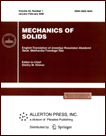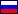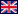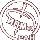Mechanics of SolidsA Journal of Russian Academy of Sciences Founded in January 1966 Issued 6 times a year Print ISSN 0025-6544Online ISSN 1934-7936RussianEnglish About Journal | Issues | Guidelines | Editorial Board | Contact UsWeb hosting is providedby the Ishlinsky Institute forProblems in Mechanicsof the RussianAcademy of Sciences
IssuesArchive of Issues2010-5pp.743-756

# Archive of Issues

 Total articles in the database: 9179 In Russian (Изв. РАН. МТТ): 6485 In English (Mech. Solids): 2694
<< Previous article | Volume 45, Issue 5 / 2010 | Next article >>
E.M. Nekislykh and V.I. Ostrik, "Problems on Elastic Equilibrium of a Wedge with Cracks on the Axis of Symmetry," Mech. Solids. 45 (5), 743-756 (2010)
Year 2010 Volume 45 Number 5 Pages 743-756
DOI 10.3103/S0025654410050109
Title Problems on Elastic Equilibrium of a Wedge with Cracks on the Axis of Symmetry
Author(s) E.M. Nekislykh (Makarenko Sumy State Pedagogical University, Romenskaya St., 87, Sumy, 40002 Ukraine)
V.I. Ostrik (Institute of Applied Physics, National Academy of Sciences of Ukraine, Petropavlovskaya St., 58, Sumy, 40030 Ukraine, ostrik_v@rambler.ru)
Abstract We use the Wiener-Hopf method to obtain exact solutions of plane deformation problems for an elastic wedge whose lateral sides are stress free and which has rectilinear cracks on its axis of symmetry. In problem 1, a finite crack issues from the wedge apex edge; in problem 2, a half-infinite crack originates at a certain distance from the wedge apex edge; and in problem 3, the wedge contains an internal finite crack.

Earlier, many authors obtained approximate solutions to these problems [1-10] and exact solutions to problem 1 [11-17] and homogeneous problem 2 [18-20] (see also ).

The method of approximate conformal transformation [1, 2] and that of integral equations [3, 4] were used to study a specific case of problem 1 about equilibrium of an elastic half-plane with a boundary crack perpendicular to the half-plane boundary. The solution to problem 1 was obtained in  by reducing the problem to the Riemann problem for analytic functions and in [6, 7], by the Wiener-Hopf method. In , the problem coefficient was factorized in terms of Cauchy-type integrals, but no further calculations were presented, and in [6, 7], an approximate factorization was performed by approximating the factorized function. In , problems 1 and 2 were reduced to Fredholm integral equations of the second kind, which were solved numerically. In problem 1, values of the integral equation density were calculated, and the normal displacements of the crack edges were expressed in terms of this density in the form of Abel integrals; no calculations were given in problem 2.

The exact values of the stress intensity factors in problem 1 were obtained in [12-17] by the Wiener-Hopf method. The paper  considered the case of linear normal stresses given on the crack edges in the absence of tangential stresses was considered, the papers [13-16], the case of concentrated forces acting on the crack edges, and the paper , the case of concentrated moments applied to the wedge apex.

Solutions to the homogeneous problem 2, where the crack edges are stress free and the principal vector and the principal moment of stresses are given at infinity, were constructed by the Wiener-Hopf method in [18-20].

Problem 3 was studied in [6, 7, 9] by different approximate methods: using the asymptotic solution of the integral equation, solving the Fredholm integral equation of the second kind by the method of successive approximations after approximating the Fourier transform of the difference kernel of the original integral equation, and using another approximation of the kernel to reduce the problem to a singular integral equation admitting a solution in closed form. Here, the asymptotic method can be used only in the case of a crack relatively distant from the wedge apex, and the approximation of the kernel transform gives satisfactory results if the angle at the wedge apex exceeds π. The authors present the numerical results for the stress intensity factors and the displacement jump at an internal point of the crack in the case where the wedge is a half-plane. In [6-9], the cases of rigid clamping and hinged support of the faces of an elastic wedge were investigated. Problem 3 was numerically solved by the method of singular integral equations in , where the case of fixed sides of the wedge was also considered.

In what follows, problems 1 and 2 whose integral equations are given on a half-infinite interval and have distinct kernels are solved by the Wiener-Hopf method , and problem 3 is solved by the generalized Wiener-Hopf method, developed in [23-25] for solving integral equations with difference kernel on a finite interval. The coefficient of the functional Wiener-Hopf equation is factorized in terms of infinite products. We also present numerical results for the stress intensity factors, the normal stress distribution on the crack continuation line, and the normal displacements of the crack edges.
Keywords elastic wedge, crack, stresses, Wiener-Hopf method, factorization
References
 1 L. A. Wigglesworth, "Stress Distribution in a Notched Plate," Math. 4 (7), 76-96 (1957). 2 O. L. Bowie, "Rectangular Tensile Sheet with Symmetric Edge Cracks," Trans. ASME. Ser. E. J. Appl. Mech. 31 (2), 208-212 (1964). 3 G. R. Irwin, The Crack-Extension Force for a Crack at a Free Surface Boundary, Report No. 5120 (Naval Research Lab., 1958). 4 O. L. Bowie and D. M. Neal, "Single Edge Cracks in Rectangular Tensile Sheet," Trans. ASME. Ser. E. J. Appl. Mech. 32 (3), 708-709 (1965). 5 J. E. Sraeley and B. Gross, "Stress Intensity Factors for Crackline-Loaded Edge-Crack Specimens," Mat. Res. Stand. 7 (4), 155-162 (1967). 6 B. I. Smetanin, "Certain Problems of Cracks in Elastic Wedge and Plate," Inzh. Zh. MTT, No. 2, 115-122 (1968) [Mech. Solids (Engl. Transl.)]. 7 B. I. Smetanin, "On a Mixed Problem of Elasticity Theory for a Wedge," Prikl. Mat. Mekh. 32 (4), 708-714 (1968) [J. Appl. Math. Mech. (Engl. Transl.) 32 (4), 732-739 (1968)]. 8 R. P. Srivastav and Prem Narain, "Certain Two-Dimensional Problems of Stress Distributions in Wedge-Shaped Elastic Solids under Discontinuous Load," Proc. Cambridge Phil. Soc. 61 (4), 945-954 (1965). 9 V. M. Alexandrov, B. I. Smetanin, and B. V. Sobol', Thin Stress Concentrators in Elastic Bodies (Fizmatlit, Moscow, 1993) [in Russian]. 10 O. Tamate and T. Kondo, "Stress Singularities around a Crack in an Elastic Wedge," Trans. Jap. Soc. Mech. Engng 44 (379), 756-761 (1978). 11 R. D. Bantsuri, "The Solution of the First Fundamental Problem in the Theory of Elasticity for a Wedge Having a Finite Slot," Dokl. Akad. Nauk SSSR 167 (6), 1256-1259 (1966) [Sov. Math. Dokl. (Engl. Transl.) 11 (4), 359-361 (1966)]. 12 H. E. Doran, "The Wedge with a Symmetric Crack at the Vertex in Plane Electostatics," J. Inst. Math. Appl. 5 (4), 363-372 (1969). 13 A. A. Khrapkov, "An Infinite Triangular Wedge with a Cut on Its Bisectrix under the Action of Concentrated Forces Applied to the Cut Edges," Izv. Akad. Nauk SSSR. Mekh. Tverd. Tela, No. 5, 88-97 (1972) [Mech. Solids (Engl. Transl.)]. 14 F. Quchterlony, "Symmetric Cracking of a Wedge by Concentrated Load," Int. J. Engng Sci. 15 (2), 109-116 (1977). 15 F. Quchterlony, "Some Stress Intensity Factors for Self-Similar Cracks, Derived from Path-Independent Integrals," J. Elasticity 8 (3), 259-271 (1978). 16 S. F. Stone and R. A. Westmann, "Stress Intensity Factors for Cracked Wedges," Int. J. Solids Struct. 17 (3), 345-358 (1981). 17 R. D. Gregory, "The Edge-Cracked Circular Disc under Symmetric Pin-Loading," Math. Proc. Cambridge Phil. Soc. 85 (3), 523-538 (1979). 18 A. E. Sadykhov, "Cracked Wedge," Dep. VINITI AN SSSR 28.03.79 No. 1091 (Azerb. Gos. Ped. Inst., Baku, 1979) [in Russian]. 19 L. A. Kipnis, "Elastic Equilibrium of a Wedge with a Crack," Prikl. Mat. Mekh. 43 (1), 153-159 (1979) [J. Appl. Math. Mech. (Engl. Transl.) 43 (1), 164-170 (1979)]. 20 A. E. Sadykhov, "Elasticity-Theory Problem for Wedge with Semiinfinite Crack Subjected to Point Moment," Prikl. Mekh. 16 (5), 91-96 (1980) [Int. Appl. Mech. (Engl. Transl.) 18 (5), 431-437 (1980)]. 21 M. P. Savruk, Stress Intensity Factors in Cracked Bodies, in V. V. Panasyuk (Editor) Fracture Mechanics and Strength of Materials, Vol. 2 (Naukova Dumka, Kiev, 1988) [in Russian]. 22 B.  Noble, Methods Based on the Wiener-Hopf Technique for the Solution of Partial Differential Equations (Pergamon Press, London etc., 1958; Izd-vo Inostr. Lit., Moscow, 1962). 23 M. P. Ganin, "On a Fredholm Integral equation whose Kernel Depends on the Difference of the Arguments," Izv. Vyssh. Uchebn. Zaved. Mat., No. 2, 31-43 (1963). 24 M. M. Ignatenko and V. Kh. Kirillov, "On the Solution of Some Problems of Mathematical Physics," Differents. Uravn. 5 (7), 1296-1302 (1969) [Differ. Equations (Engl. Transl.)]. 25 Yu. A. Antipov, "Exact Solution of the Problem on the Indentation of an Annular Die into a Half-Space," Dokl. Akad. Nauk Ukr. SSR Ser. A Fiz. Mat. Tekhn. Nauki, No. 7, 29-33 (1987). 26 V. I. Ostrik and A. F. Ulitko, Wiener-Hopf Method in Contact Problems of Elasticity (Naukova Dumka, Kiev, 2006). 27 M. V. Fedoryuk, Asymptotics: Integrals and Series (Nauka, Moscow, 1987) [in Russian]. 28 G. P. Cherepanov, Mechanics of Brittle Fracture (Nauka, Moscow, 1974) [in Russian].If you find a misprint on a webpage, please help us correct it promptly - just highlight and press Ctrl+Enter
 101 Vernadsky Avenue, Bldg 1, Room 246, 119526 Moscow, Russia • (+7 495) 434-3538 • mechsol@ipmnet.ru • https://mtt.ipmnet.ru Founders: Russian Academy of Sciences, Branch of Power Industry, Machine Building, Mechanics and Control Processes of RAS, Ishlinsky Institute for Problems in Mechanics RAS © Mechanics of Solids webmaster